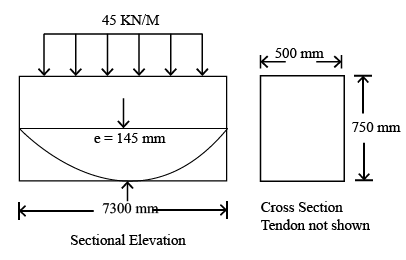# ISRO Scientist or Engineer Civil 2014

Instructions

For the following questions answer them individually

Question 61

# The most common coagulantisQuestion 62

# Self cleansing velocity isQuestion 63

# When design imposed load doesnot exceed three-fourths of the design dead load,the load arrangement on structural frame as per IS 456-2000 isQuestion 64

# A rectangular column section of $$250 \times 400$$ mm reinforced with 6 steel bars of $$Fe_{500}$$, each of 25mm diameter. Concrete Mix is $$M_{30}$$. Axial load on column section with minimum eccentricity as per IS 45 6-2000using limit state method can be applied upto:Question 65

# The effective length of circular electrical pole of length L and constant diameter erected on groundis :Question 66

# If $$\psi$$ is the nominal diameterofreinforcing bar, $$f_{x}$$, is compressive stress in the bar and $$f_{bd}$$ is design bondstress of concrete,the anchorage length $$l_{a}$$ of straight bar in compression is equalto:Question 67

# A concrete beam prestressed with a parabolic tendon is shown in the sketch. The eccentricity of the tendon is measured from the centroid of the cross section. The applied prestressing force at service is 1620 KN. The uniformly distributed load of $$\frac{45KN}{m}$$ includes self weight.The stress (in $$\frac{N}{mm^{2}}$$) in the bottom fibre at Mid Span isQuestion 68

# As per IS 456-2000; in the limit state design of a flexural member, the strain in reinforcing bar undertension at ultimate state should not be less thanQuestion 69

# As per Indian Standard code of practice for prestressed concrete (IS 1343 — 1980), the minimum grade of concrete to be used for post tensioned and prestressed. structuralelements are respectivelyQuestion 70

# Thefinal deflection of a structure due to all loads including the effects of temperature, creep and shrinkage and measured from the as — cast level of support of floor, roofsand all other horizontal forces should not normally exceedOR